# SSC CGL 2014 Tier 1 27 April shift 8

Instructions

For the following questions answer them individually

Question 191

Identify the region that represents students studying Biology and Computer not Mathematics.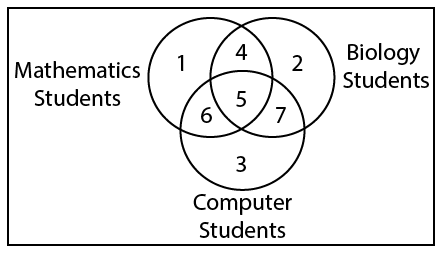Question 192

Find out the number of triangles in this figure.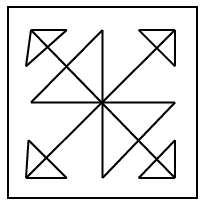Question 193

Which of the following diagrams represents the correct relationship between Books, Novels and Dictionaries ?

Question 194

If the difference between the no. of dots on the opposite faces is 3, find out the figure which is correct ?

Instructions

In each of the following questions, which answer figure will
complete the pattern in the question figure ?

Question 195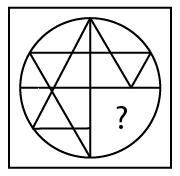Question 196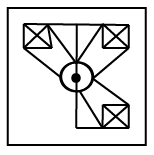Instructions

For the following questions answer them individually

Question 197

Which of the answer figures is , exactly the water image of the given figure ?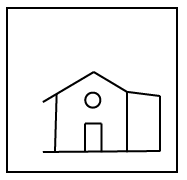Question 198

From the given answer figures, select the one which is hidden/ embedded, in the question figure?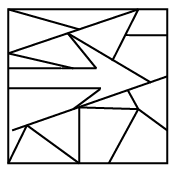Question 199

A piece of paper is folded and cut as shown below in the question figures. From the given answer figures, indicate how it will appear when opened.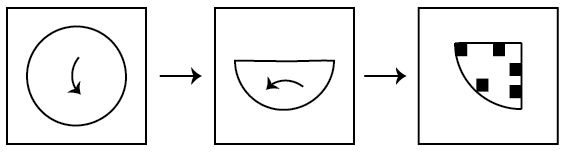Question 200

A word is represented by only one set of numbers as given in any one of the alternatives. The sets of numbers given in the alternatives are represented by two classes of alphabets as in two matrices given below. The columns and rows of Matrix I are numbered from 0 to 4 and that of Matrix II are numbered from 5 to 9. A letter from these matrices can be represented first by its row and next by its column. e.g., ‘M’ can be represented by 42, 31. etc. and ’13’ can be represented by 95, 88 etc. Similarly, you have to identify the set for the word given ‘ROST.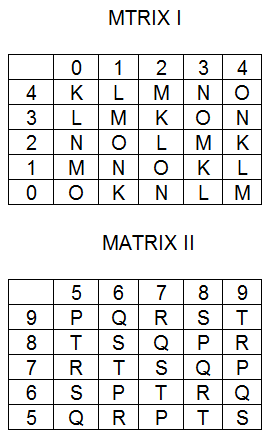OR